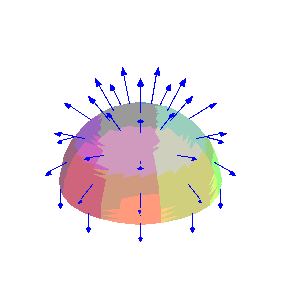Function: surfarrows - plot a 3D surface (parametric or cartesian) with arrows from a vector field at points on the surface.

Calling sequence:

surfarrows(fld,surf,s=a1..b1,t=a2..b2);
Parameters:

fld - the vector field, either a 3-component list or vector expression in the parameters s and t (specifying the vectors in terms of those parameters) or a function of three variables whose values are 3-component lists or vectors (specifying the vector field in terms of the spatial coordinates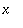,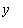and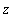).

surf - the surface, either a 3-component list or vector expression in the parameters s and t (for a parametric representation of the surface) or a scalar expression in s and t (representing thecoordinate while s and t are theandcoordinates respectively).

s , t - names for the parameters.

a1 , b1 , a2 , b2 - endpoints for the parameter intervals. a1 and b1 must be constants, while a2 and b2 may depend on the first parameter s .

Optional arguments:

arrowgrid= [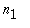,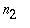] for arrows in anbygrid, equally spaced in terms of the parameters of the surface (default is arrowgrid=[8,8] ).

scalefactor=to multiply arrow lengths by real constant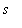.

arrowthickness=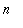for thickness of the arrow shaft (should be 0, 1, 2 or 3, default is the same as the thickness option if specified, or 3 if not).

arrowcolour=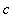to specify arrow colour (default is the same as the colour option if specified, or red if not). The alternate spelling arrowcolor is allowed.

other 3D surface plotting options.

Description:

This function creates a 3D plot data object which consists of a surface, plotted as with the command
plot3d(surf, s=a1..b1, t=a2..b2) (including all options of the surfarrows command except arrowgrid , scalefactor , arrowthickness and arrowcolour ), plus arrows representing the vector field fld drawn at points on the surface.

Unless the option scaling=constrained ("Projection, Constrained" on the plot menu or "1-1" on the context bar) is used, the shapes of the arrowheads may be distorted.

This function is part of the package surfarro in the Maple Advisor Database library, and must be loaded before use by the command with(surfarro); or with(surfarro,surfarrows); .

Examples:

> with(surfarro,surfarrows):

Surface is a sphere represented parametrically, vectors are tangent to meridians.

> surf1:= [sin(t)*cos(s),sin(t)*sin(s),cos(t)];
F1:= diff(surf1,t);
surfarrows(F1, surf1, s = 0 .. 2*Pi, t = 0 .. Pi,
scalefactor=0.4, style=patch, scaling=constrained, orientation=[0,70]);

`                    surf1 := [sin(t) cos(s), sin(t) sin(s), cos(t)]`

`                     F1 := [cos(t) cos(s), cos(t) sin(s), -sin(t)]`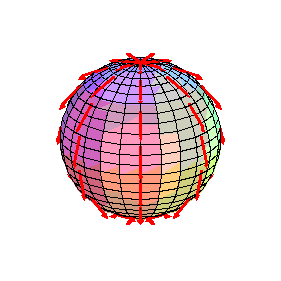Surface is a hemisphere represented in Cartesian coordinates, vectors are normal to surface. Note that theinterval depends on.

> surf2:= sqrt(1-x^2-y^2);
F2:= [x,y,surf2];
surfarrows(F2,surf2, x = -1 .. 1, y = -0.999*sqrt(1-x^2) .. 0.999*sqrt(1-x^2), orientation = [90, 45],
scalefactor=1/3, style=patchcontour, scaling=constrained, arrowthickness = 2);

```                                                  2    2
surf2 := sqrt(1 - x  - y )```

```                                                   2    2
F2 := [x, y, sqrt(1 - x  - y )]```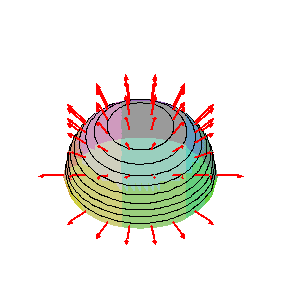Parametric hemisphere, vector field is a function of,andcoordinates (a dipole field in this case):

> F3:= (x,y,z) -> [3*x*z, 3*y*z, 2*z^2-x^2-y^2];
surfarrows(F3,surf1, s = 0 .. 2*Pi, t = 0 .. Pi/2,
arrowcolour=blue, scalefactor = 1/4, arrowgrid=[10,5], arrowthickness=1, scaling=constrained, style=patchnogrid, orientation=[0,60]);

```                                                        2    2    2
F3 := (x, y, z) -> [3 x z, 3 y z, 2 z  - x  - y ]```